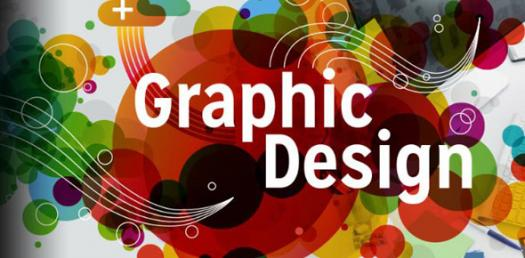# Graphic Design Standards: Theatre

10 Questions | Total Attempts: 218SettingsIdentify the following graphics as they relate to drafting for the theatre. You may use the following link to review the information

• 1.
What does the following graphic represent?
• A.

Plate Border

• B.

Dimension Line

• C.

Visible Outline

• D.

• 2.
What does the following graphic represent?
• A.

Dimension Line

• B.

Plaster Line

• C.

Center Line

• D.

• 3.
What does the following graphic represent?
• A.

Dimension Line

• B.

Plaster Line

• C.

Center Line

• D.

• 4.
What does the following graphic represent?
• A.

Dimension Line

• B.

Plaster Line

• C.

Center Line

• D.

• 5.
What does the following graphic represent?
• A.

Dimension Lines

• B.

• C.

Page Border

• D.

Center Line

• 6.
1/2" = 1' Scale indicates that every 1/2" equals 1'. A two inch line drawn on a design would be equal to___?
• A.

2'

• B.

4'

• C.

8'

• D.

12'

• 7.
Using 1/4" scale where 1/4" = 1', a two inch  line drawn in a design would be equal to___?
• A.

2'

• B.

4'

• C.

8'

• D.

12'

• 8.
The following flat built from 1 x 4 would indicate that the overall dimensions are 4' x 8'. What isn't readily visible that is required to construct this flat?
• A.

Assembly details

• B.

Individual Component Dimensions

• C.

Color

• D.

A and B

• 9.
Based on your experience with the construction of 4 x 8 flats built from 1 x 4, which part of the flat would be 7' - 10 1/2"?
• A.

Rail

• B.

Stile

• C.

Toggle

• D.

Skin

• 10.
Based on your experience with the construction of 4 x 8 flats built from 1 x 4, which part of the flat would be 3' - 10 1/2"?
• A.

Rail

• B.

Stile

• C.

Toggle

• D.

Skin

Related TopicsBack to top